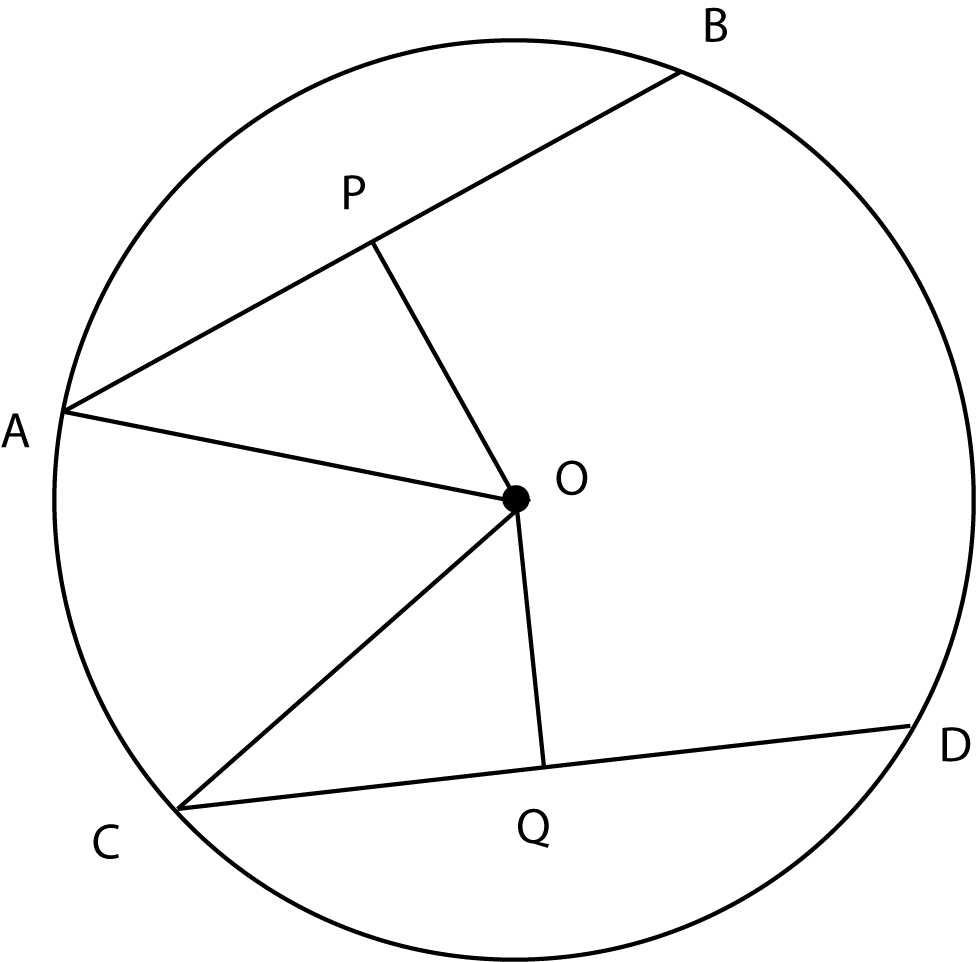### Prove: AP = CQAP + AP = CQ + CQ
Substitution
Substitution
AP + PB = CQ + QD
If a diameter is perpendicular to a chord, it bisects the chord
Equal arcs have equal chords
AB = AP + PB and CD = CQ + QD
Definition
Algebra
AP = CQ
OP bisects AB and OQ bisects CD
OP is perpendicular to AB and OQ is perpendicular to CD
AP = PB and CQ = QD
AB = CD
Given